﻿ Influence of Hole Deviation on the Reliability of Ore ReserveInternational Journal of Mining Engineering and Mineral Processing

p-ISSN: 2166-997X    e-ISSN: 2166-9988

2022;  11(1): 1-7

doi:10.5923/j.mining.20221101.01

Received: Dec. 20, 2021; Accepted: Jan. 4, 2022; Published: Apr. 15, 2022### Influence of Hole Deviation on the Reliability of Ore Reserve

Nwosu J. I., Amadi K.

Department of Geology, University of Port Harcourt, Nigeria

Correspondence to: Nwosu J. I., Department of Geology, University of Port Harcourt, Nigeria.
 Email: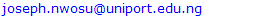Abstract

Hole deviation from the designed trajectory gives a false thickness of ore bodies. Consequently the reserve of a mineral deposit estimated based on this false thickness is liable to differ from the true reserve of the deposit. There is therefore the need to estimate the level of reliability of ore reserve based on deviation angles during exploration. In this research, geometrical modelling of ore bodies of a mineral deposit has been used to estimate the true and false reserve of a mineral deposit. The standard deviation and coefficient of variation of the false reserve from the true reserve at various angles of deviation were also estimated. Based on coefficient of variation a mathematical model has been suggested for estimating the reliability of ore reserve for various angles of deviation during exploratory drilling operations.

Keywords: False reserve, True reserve, Coefficient of variation, Reliability, Confidence level

Cite this paper: Nwosu J. I., Amadi K., Influence of Hole Deviation on the Reliability of Ore Reserve, International Journal of Mining Engineering and Mineral Processing , Vol. 11 No. 1, 2022, pp. 1-7. doi: 10.5923/j.mining.20221101.01.

### 1. Introduction

Borehole deviation is a shift of the hole during drilling from the initially designed trajectory. If the initial trajectory of the hole is to be vertical, for instance during the investigation of a horizontally bedding deposit and hole begins to drift away from the vertical trajectory, this curvature is most likely to give a false impression of the thickness of the deposit. On the other hand, if a horizontal borehole is desired to be drilled to confirm the thickness of an ore body in an exploration programme, a shift from a horizontal trajectory will be regarded as a deviation. In our case which is the investigation of the thickness of ore bodies of steeply dipping deposit, hole deviation is made use of to ascertain the thickness of ore bodies. In fact the exploratory hole is given an initial angle of deviation to enable it move in such a trajectory as to intersect the ore bodies at 90°. This should give the true thickness of ore body. However, in practice, no matter how precise the estimation tool is and no matter how detailed the study of the rock lithology is done, the exploratory hole usually deviates from the designed trajectory. This deviation is influenced by the variation of rock mechanical properties and expertise in borehole orientation and technology. (Alwyn et’al 1991) noted that hole deviation from design trajectory is one of the factors affecting the accuracy of ore reserve. They further stated that those who estimate ore reserves do not accompany it with level of reliability of the ore reserve. The impact of variability of ore body thickness, ore density and grade on project NPV in a gold mine has been demonstrated by Grant (2014). The risk imposed by reserve reliability on mineral project viability is widely acknowledged by official organs of government on mineral resource management, hence Canadian Institute of Mining and Metallurgy (2019) devotes much attention to risk analysis in reserve estimation and classification with the goal of assisting mineral practitioners to establish mineral resource confidence. Thus creation of awareness on the level of reliability of ore reserve can assist mine planners to make informed decisions on mine project’s NPV (net present value) and IRR (internal rate of return).
The reliability of ore reserve is the probability that the ore reserve figure provided during estimation process will be available in the deposit when the project comes on stream. In order to ensure a balanced view on mineral project reporting, it is necessary to report both on the opportunities a mineral project presents as well as the inherent uncertainties associated with the reserve figure (Noppe 2014). All these tend to show that more work has to be done in the area of reserve reliability estimation.

### 2. Methodology

The process of developing a model for reliability estimation of ore reserve is being proposed here through a geometrical modelling process in which the mineral ore body model is used. The ore body model is divided into grids of 50m each, depths are given to the ore body at each profile and the thickness of the ore body is also measured.
The reserve of ore body between two profiles is given as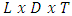(1)
Where
L = Distance between two profiles 50m
D = average depth of ore body between two profiles
T = Average thickness of ore bodies between two profiles
Because of complex configuration of ore bodies, the average thickness between two profiles is given by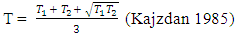(2)
Where
T1 = Thickness of ore body along the first profile
T2 = Thickness of ore body along the second profile
Then a deviation of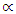degrees is given to the borehole trajectory and apparent thickness is calculated using the following formular.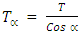(3)
Where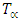= False (apparent) thickness of ore body when the angle of deviation is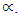The apparent thicknesses caused bydegrees (= 10°, 15°, 20°, 25° and 30°) are now used to recalculate ore reserve for each deviation angle and all the ore reserves are tabulated. The total reserve for each angle of deviation and for the true reserve (the reserve without deviation) are obtained by summing up the reserve in all the profiles covering the entire length of ore body. The reserves are expressed in millions of tons.

#### 2.1. Confidence Level versus Reliability

The confidence level of ore reserve is associated with the estimation of confidence interval (upper and lower limits) in which that confidence level exists. The wider the confidence interval, the higher the confidence level. In fact the confidence level is highest (100%) when the confidence interval ranges from zero to infinity. The implication of this is that confidence level does not give us the probability that the reserve estimate is correct but merely gives us the probability that the ore reserve lies within the interval (upper and lower limit). As correctly stated by Alwyn (1991), a wide confidence limit means low confidence or not assigned (Mineral deposit evaluation, page 176).
Confidence interval for a given confidence level is estimated from student’s distribution as follows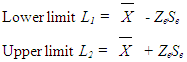(4)
Where
L1 = Lower limit of the interval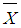= Mean deviation
Ze = the variable from student distribution based on the chosen confidence level.
Se = Standard Error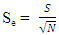(5)
Where
S = Standard deviation
N = No of samples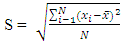(6)
The upper and lower limit of the reserve and corresponding level of confidence for various angles of deviation are tabulated.
From the foregoing, it therefore becomes clear that in order to assess the trueness of ore reserve, the reliability of ore reserve is more applicable. The reliability of ore reserve figure tells us the probability that the ore reserve predicted is close to the true reserve.
The major factor separating the false reserve from the true reserve is reflected in coefficient of variation of the false reserve from the true reserve. Coefficient of variation is estimated from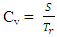(7)
Where
Cv = Coefficient of variation of false reserve from the true reserve
S = Standard deviation of false reserve from the true reserve
Tr = True reserve
Consequently reliability of ore reserve can be estimated from the following equation.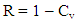(8)
Where
R = Reliability of ore reserve (in fraction) or in percentage
R = 100 (1 – Cv)
Finally the reliability of ore reserve is calculated for each range of angle of deviation and a graph in reliability and angle of deviation is plotted.

### 3. Results and Discussion

#### 3.1. Geometrical Modelling for Ore Reserve Estimation

In order to carry out geometrical modelling that will provide the relevant parameters for reliability estimation, a geometrical model of the ore body is used (fig 1). As can be shown in the figure distance between two profiles is 50m, depths of the ore body at the profiles are given in column 2 of table 1. The true thicknesses of the ore body are measured and tabulated in column 3 of table 1. Furthermore, using equation (3) the thicknesses of ore bodies at various angles of deviation (10° – 30°) were estimated and tabulated in columns 4, 5, 6, 7 & 8 of same table 1.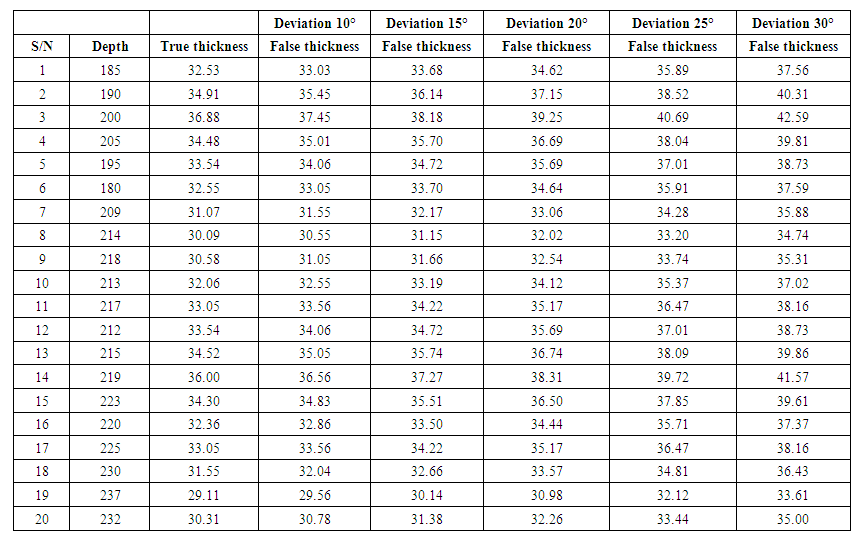Table 1. Thickness of the ore body in relation to angle of deviation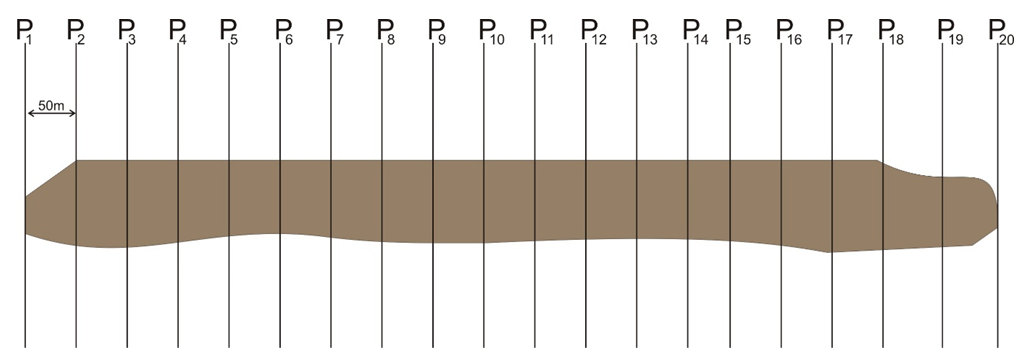Figure 1. Profiles along the strike of the ore body
To estimate the average thicknesses of ore bodies between two profiles, formula (2) was used for both true and false thicknesses and were tabulated accordingly in table 2 (Columns 3, 5, 7, 9, 11, 13). Using equation (1) the reserves of the ore body between two successive profiles were calculated for both true and false reserves and presented in columns 4, 6, 8, 10, 12 & 14 of table 2.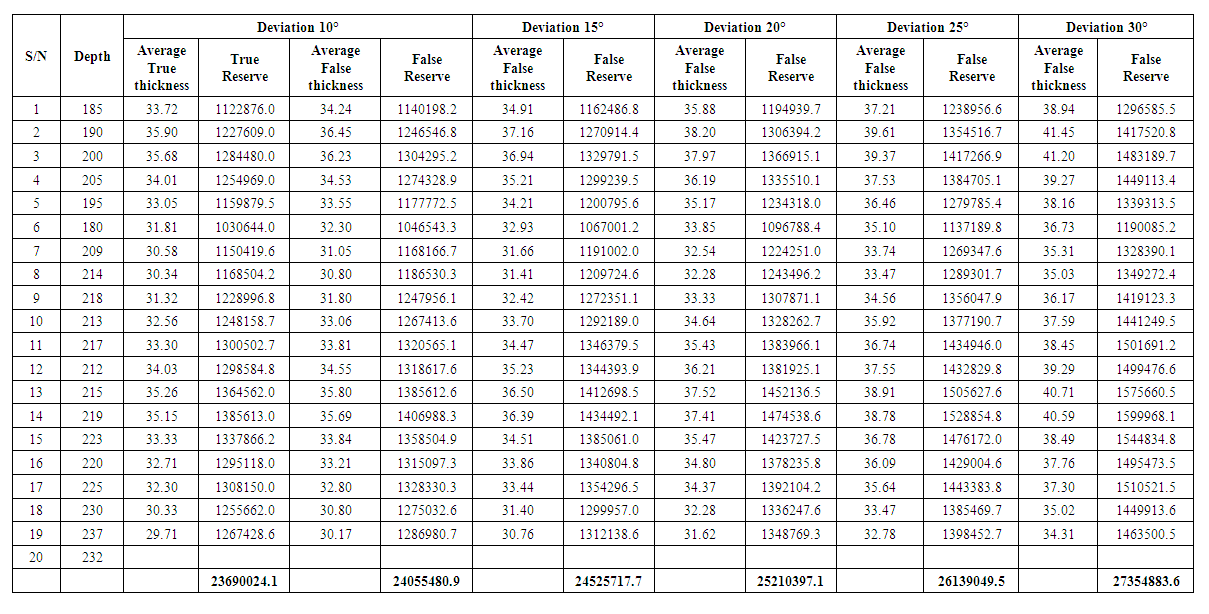Table 2. Reserve of the ore body in relation to angles of deviation
Finally the reserves in all the profile intervals for both true and false reserves are added respectively for the entire length of the ore body to get the reserve of the entire ore body.

#### 3.2. Confidence Interval, Confidence Level and Reliability Estimation

Now using the true and false reserves estimated above the confidence intervals based on the chosen confidence level are now estimated according to the various angles of deviation. In this analysis the true reserve will serve as mean reserve. This is because every member or component of a distribution revolves around the mean just as every false reserve (whether higher or lower than the true reserve) revolves around the true reserve. This approach was applied by Barry and Whateley 1995 in relation to true grade and mean grade.
For 10° deviation, standard deviation
S1 =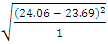= 0.37mt
Standard Error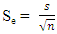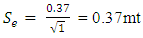To estimate Ze in formula (4) using student distribution table.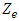= 31.82 (for 99% confidence level and 1 degree of freedom)
Mean Reserve (true reserve) = 23.69mt
Lower Limit = 23.69 – (31.82 x 0.37) = 11.92mt
Upper Limit = 23.69 + (31.82 x 0.37) = 26.03mt
Therefore Confidence interval for 99% confidence level = 11.92mt — 35.46mt
95% confidence level and 1 degree of freedom
Lower Limit = 23.69 – (6.31 x 0.37) = 21.35mt
Upper Limit = 23.69 + (6.31 x 0.37) = 26.03mt
Confidence interval for 99% confidence level = 21.35mt — 26.03mt
90% confidence level and 1 degree of freedom
Lower Limit = 23.69 – (3.08 x 0.37) = 22.55mt
Upper Limit = 23.69 + (3.08 x 0.37) = 24.83mt
Confidence interval for 90% confidence level = 22.55mt — 24.83mt
80% confidence level and 1 degree of freedom
Lower Limit = 23.69 – (1.376 x 0.37) = 23.18mt
Upper Limit = 23.69 + (1.376 x 0.37) = 24.2mt
Confidence interval for 80% confidence level = 23.18mt — 24.2mt
75% confidence level and 1 degree of freedom
Lower Limit = 23.69 – (1.0 x 0.37) = 23.32mt
Upper Limit = 23.69 + (1.0 x 0.37) = 24.06mt
Confidence interval for 75% confidence level = 23.32mt — 24.06mt
70% confidence level and 1 degree of freedom
Lower Limit = 23.69 – (.727 x 0.37) = 23.42mt
Upper Limit = 23.69 + (.727 x 0.37) = 23.96mt
Confidence interval for 70% confidence level = 23.42mt — 24.96mt
60% confidence level and 1 degree of freedom
Lower Limit = 23.69 – (.325 x 0.37) = 23.57mt
Upper Limit = 23.69 + (3.08 x 0.37) = 23.81mt
Confidence interval for 60% confidence level = 23.57mt — 23.81mt
55% confidence level and 1 degree of freedom
Lower Limit = 23.69 – (.158 x 0.37) = 23.63mt
Upper Limit = 23.69 + (.158 x 0.37) = 23.75mt
Confidence interval for 55% confidence level = 23.63mt — 23.75mt
10° - 15°
S1 =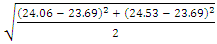= 0.65mt
Standard Error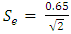= 0.46mt
Mean Reserve = 23.69mt
99% confidence level and 2 degree of freedom)
Lower Limit = 23.69 – (6.96 x 0.46) = 20.49mt
Upper Limit = 23.69 + (6.96 x 0.46) = 26.89mt
Confidence interval for 99% confidence level = 20.49mt — 26.89mt
95% confidence level and 2 degree of freedom
Lower Limit = 23.69 – (2.92 x 0.46) = 22.35mt
Upper Limit = 23.69 + (2.92 x 0.46) = 25.03mt
Confidence interval for 95% confidence level = 22.35mt — 25.03mt
90% confidence level and 2 degree of freedom
Lower Limit = 23.69 – (1.89 x 0.46) = 22.82mt
Upper Limit = 23.69 + (1.89 x 0.46) = 24.56mt
Confidence interval for 90% confidence level = 22.82mt — 24.56mt
80% confidence level and 2 degree of freedom
Lower Limit = 23.69 – (1.061 x 0.46) = 23.2mt
Upper Limit = 23.69 + (1.061 x 0.46) = 24.18mt
Confidence interval for 80% confidence level = 23.2mt — 24.18mt
75% confidence level and 2 degree of freedom
Lower Limit = 23.69 – (.816 x 0.46) = 23.31mt
Upper Limit = 23.69 + (.816 x 0.46) = 24.07mt
Confidence interval for 75% confidence level = 23.31mt — 24.07mt
70% confidence level and 2 degree of freedom
Lower Limit = 23.69 – (.617 x 0.46) = 23.41mt
Upper Limit = 23.69 + (.617 x 0.46) = 23.97mt
Confidence interval for 70% confidence level = 23.41mt — 24.97mt
60% confidence level and 2 degree of freedom
Lower Limit = 23.69 – (.289 x 0.46) = 23.56mt
Upper Limit = 23.69 + (.289 x 0.46) = 23.82mt
Confidence interval for 60% confidence level = 23.56mt — 23.82mt
55% confidence level and 2 degree of freedom
Lower Limit = 23.69 – (.142 x 0.46) = 23.62mt
Upper Limit = 23.69 + (.142 x 0.46) = 23.76mt
Confidence interval for 55% confidence level = 23.62mt — 23.76mt.
The same process is used to compute confidence interval for chosen confidence level at other angles of deviation (10 – 20, 10 – 25, 10 – 30) and are presented in table 3.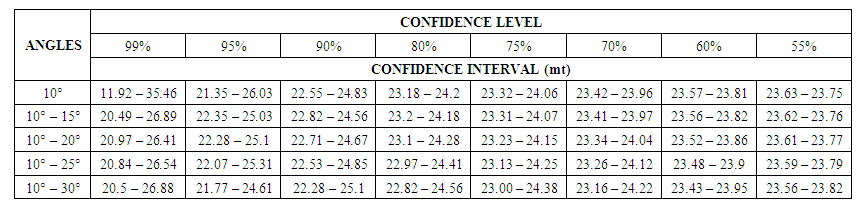Table 3. Confidence levels, confidence intervals at various angles of deviation
Coefficient of variation and Reliability Calculation
10°
Cv =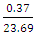= 0.02
Reliability (R) = 1 – Cv
R = 1 – 0.02 = 0.98(98%)
10°, 15°
Cv =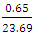= 0.03
R = 1 – 0.03 = 0.97(97%)
10°, 15°, 20°
Cv =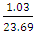= 0.04
R = 1 – 0.03 = 0.96(96%)
10°, 15°, 20°, 25°
Cv =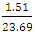= 0.06
R = 1 – 0.03 = 0.94(94%)
10°, 15°, 20°, 25° 30°
Cv =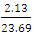= 0.09
R = 1 – 0.03 = 0.91(91%)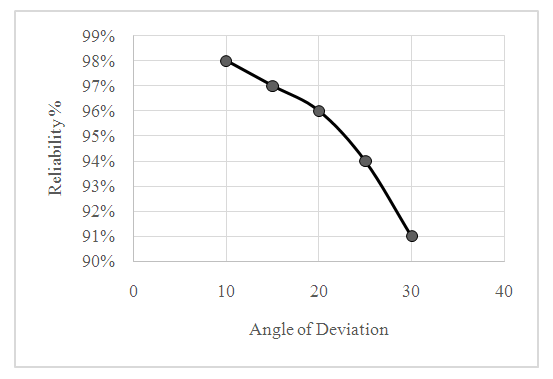Figure 2. Graph showing the relationship between angle of deviation of exploratory boreholes and the reliability of ore reserve
In order to estimate the reliability of ore reserve the exploration geologist does not need to carry out the geometrical modelling as shown above, because coefficient of variation depends only on the angles of deviation and not on the reserves of the deposit (Murray and Larry 2008). Consequently knowing the angle of deviation of exploratory trajectories from 90° to the ore body all that is required for reliability estimation is to pick the coefficient of variation for the corresponding angle of deviation and then calculate reliability by formula (8).
An important advantage of this reliability approach over other parameters like precision (Barnes 1980) and confidence (Whateley 2006) is its ability to be used for considering the effect of several factors. For example if during the exploration of a mineral deposit the angle of deviation of hole trajectories from the ore body varies from 10 - 30 and the mean grade of the deposit was 36% with a standard deviation of 2% and the mean specific gravity was 3.5 with a standard deviation of 0.3, then; reliability of ore reserve on account of hole deviation is 91% (table 4). Coefficient of variation of ore grade =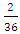= 0.056. From formular (8), reliability on the basis of grade is 1 – 0.056 = 0.94 or 94%.
 Table 4. Reliability of ore reserve based on range of angles of deviation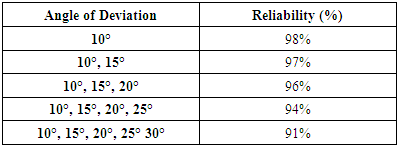For specific gravity coefficient of variation is =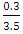= 0.09. Reliability = 1 – 0.09 = 0.91 or 91%
Therefore the reliability of the mineral property under the joint effect of the 3 factors is equivalent to: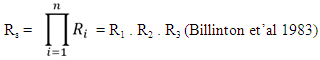Where;
R1 = the mineral deposit reliability on reserve estimation
R2 = the mineral deposit reliability grade estimation
R3 = the mineral deposit reliability on specific gravity
Rs = the joint reliability under the effects of the 3 factors
Consequently, Rs = 0.91 x 0.94 x 0.91 = 0.78 or 78%.

### 4. Discussion and Conclusions

1. Geometrical modelling approach provides an opportunity to obtain true reserve and false reserve in a multiple deviation angle exploration. Using this true and false reserve, it is then possible to estimate the standard deviation of false reserve from true reserve as well as coefficient of variation of the false reserve from true reserve.
2. The major parameter that reflects the deviation of false reserve from true reserve is coefficient of variation. Thus coefficient of variation can be used to show how close the estimated reserve is from the true reserve i.e reliability of ore reserve. Thus formula (8) provides the opportunity to estimate reliability of reserve based on angle of deviation of exploratory boreholes from 90° to the ore body during exploration.
3. Our analysis has also shown that the confidence level does not show the accuracy of ore reserve but merely shows the probability that the ore reserve lies within certain range (upper and lower limit).

### References

  Alwyn E. Annels (1991) Mineral Property Evaluation: A Practical Approach. Published by Springer, London. Pp 176.  Barry and Whateley (1995) Introduction to Mineral Exploration: Evaluation Technique. Published by Blackwell Publishing Ltd. Pp 199 – 252.  Barnes M.P. (1980) Computer Assisted Mineral Appraisal and Feasibility, Society of Mining Engineers, American Institute of Mining, Metallurgical Petroleum Engineers, New York, 167 Pp.  Billinton, R. (1983) Reliability Evaluation of Engineering Systems. Pitmans Advanced Publishing Ltd, Boston, USA, Pp 67.  CIM Estimation of Mineral Resources and Mineral Reserve Best Practice Guidelines (2019).  Grant, N (2014) An Integrated Risk Evaluation Model for Mineral Deposit. Ph.D Thesis, University of Adelaide, Australia.  Kajzdan A.B (1985), Prospecting and Exploration for Mineral Deposit. Published by Technicheskaya Kniga, Leningrad (in Russian).  Noppe, M.A (2014) Communicating confidence in Mineral Resources and Mineral Reserves, Journal of the Southern African Institute of Mining and Metallurgy, Vol.114 n.3 Johannesburg.  Murray R. Spiegel, Larry J. Stephens (2008). Theory and Problem of Statistics Fourth Edition. McGraw-Hill Companies, U.S.A. Pp 571.  Whateley (2006) Introduction to Mineral Exploration. Published by Blackwell Publishing Ltd. 481 Pp.